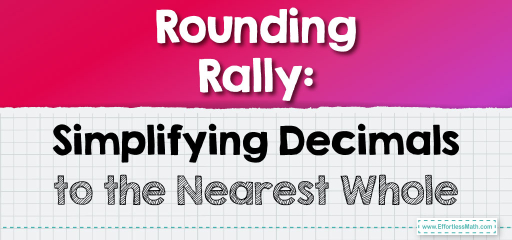# Rounding Rally: Simplifying Decimals to the Nearest Whole

Rounding decimals is a valuable skill that simplifies numbers, making them easier to work with, especially in real-world scenarios like estimating costs or measurements. The key to rounding is determining which whole number or decimal place a number is closest to. Let's dive into the steps to round decimals effectively.## Rounding Decimals

### Example 1:

Decimal to Round: $$4.58$$

Rounding to the Nearest Whole Number:

1. Look at the tenths place: $$5$$.

2. Since $$5$$ is equal to or greater than $$5$$, round up the ones place by $$1$$.

3. The tenths and other places become $$0$$.

Rounded Decimal:

$$5$$

The Absolute Best Book for 5th Grade Students

### Example 2:

Decimal to Round: $$3.42$$

Rounding to the Nearest Tenth:

1. Look at the hundredths place: $$2$$.

2. Since $$2$$ is less than $$5$$, keep the tenths place as it is.

3. The hundredths and other places become $$0$$.

Rounded Decimal:

$$3.4$$

Rounding decimals is all about simplification. Whether you’re estimating costs, measurements, or just trying to get a general idea of a value, rounding provides a more digestible number. Remember the golden rule: if the number you’re looking at is $$5$$ or above, round up; otherwise, round down. With practice, you’ll be able to round decimals in a snap!

### Practice Questions:

1. Round $$5.67$$ to the nearest whole number.

2. Round $$2.34$$ to the nearest tenth.

3. Determine the rounded value of $$7.89$$ to the nearest whole number.

4. What is $$0.505$$ rounded to the nearest tenth?

5. Round $$8.213$$ to the nearest hundredth.

A Perfect Book for Grade 5 Math Word Problems!

1. $$6$$

2. $$2.3$$

3. $$8$$

4. $$0.5$$

5. $$8.21$$

The Best Math Books for Elementary Students

### What people say about "Rounding Rally: Simplifying Decimals to the Nearest Whole - Effortless Math: We Help Students Learn to LOVE Mathematics"?

No one replied yet.

X
51% OFF

Limited time only!

Save Over 51%

SAVE $15 It was$29.99 now it is \$14.99